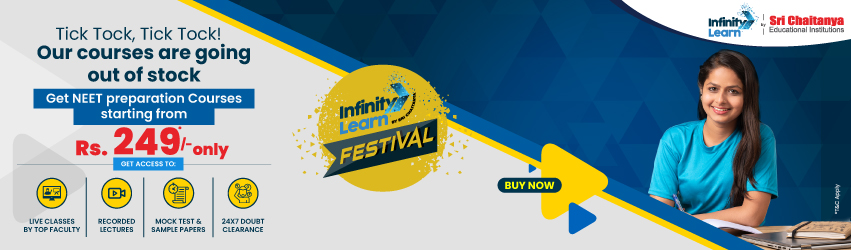NCERT Solutions for Class 12 Maths Chapter 11 Three Dimensional Geometry 11.2

# NCERT Solutions for Class 12 Maths Chapter 11 Three Dimensional Geometry 11.2Infinity Learn NEET Festival
Infinity Learn IIT JEE Festival

All 11.2 exercise questions are answered in NCERT Solutions for Class 12 Maths Chapter 11 Three Dimensional Geometry. The principals studied in Maths chapter 11 are the foundation for NCERT Solutions for Class 12 11.2 Exercise. This practice is crucial for both the CBSE Term II exam and competitive exams. To do well on your exam, download these NCERT Solutions and practice them offline.

Do you need help with your Homework? Are you preparing for Exams? Study without Internet (Offline)
×
Exercise 11.2

NCERT Solutions for Class 12 Maths Chapter 11 Three Dimensional Geometry 11.2

Need FREE NCERT/CBSE/IIT-JEE/NEET Study Material?

Using the NCERT solutions for Ex 11.2 Class 12 Maths is the best option for the CBSE students when they are preparing for their exams. This chapter includes many exercises. One of them is Exercise 11.2 whose solutions are available in pdf format on this page. You can also download these solutions online from our website or app. Infinite Learn subject matter experts have designed solutions from the exercise with the utmost care and by keeping in mind all the guidelines by CBSE. Class 12 Students which have prepared with all the exercises are given in this chapter can easily score well in the exams. By using these solutions, students came to know about the pattern of questions that are asked in the exam from this chapter and also know about the marks weightage of the chapter. So they can prepare well.

Along with this exercise, there are many number of exercises included in this chapter that contain innumerable questions as well. All these solutions are designed by subject matter experts as mentioned earlier. Hence all of these are considered as superior quality and students must refer to these solutions. To score well in the exam all these solutions must be practiced well and all other questions must be solved which are given next to it. Do not delay any more. Download the NCERT solutions for Class 12 Maths Chapter 11 Exercise 11.2 from Infinity Learn website now for better exam preparation. If you have the Infinite learn app on your phone, you should download the same through the app as well. You can use these solutions online and offline as well

1. Can I score full marks in Class 12 Chapter 11 Maths?

Ans. Yes, you can get full marks in Class 12 Maths. All it takes to score full marks in Maths is constant practice. Having all of your concepts clear in this subject is essential since many questions are expected in the board examination from this chapter. This will make you confident and well versed with the chapter and fetch you full marks in the exam. You must also refer to the NCERT Solutions for Chapter 11 of Class 12 Maths.

1. What are the main concepts on which Class 12 Maths Exercise 11.2 is based?

Ans. The Class 12 Maths Exercise 11.2 is based on Three Dimensional Geometry. The main topics which are covered in this chapter are Equation of a Line in Space, Angle between Two Lines and the shortest distance between Two Lines. The exercise contains problems on these topics and solving these will help students gain a better understanding of all the concepts of this chapter and score well in the exams.

1. Do I need to practice all the questions provided in Class 12 Maths Exercise 11.2?

Ans. Yes, students should solve all the questions in the Class 12 Maths Exercise 11.2.  To get a clear idea about the various problems from this chapter, and answer all the questions accurately, one needs regular practice. The exercise is prepared in such a way that it will help you in both these aspects. For further guidance, you can refer to NCERT Solutions for Chapter 11 Exercise 11.2 of Class 12 Maths on the Vedantu website for free of cost.

Join Infinity Learn Regular Class Programme!